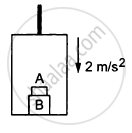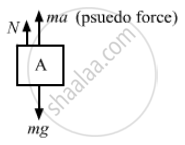# The Elevator Shown in Figure (5−E5) is Descending with an Acceleration of 2 M/S2. the Mass of the Block a is 0.5 Kg. What Force is Exerted by the Block a on the Block B? - Physics

Sum

The elevator shown in the following figure is descending with an acceleration of 2 m/s2. The mass of the block A is 0.5 kg. What force is exerted by the block A on the block B?#### Solution

When the elevator is descending, a pseudo-force acts on it in the upward direction, as shown in the figure.From the free-body diagram of block A,
$mg - N = ma$
$N = m\left( g - a \right)$
$\Rightarrow N = 0 . 5\left( 10 - 2 \right) = 4 N$
So, the force exerted by the block A on the block B is 4 N..

Concept: Newton's Third Law of Motion
Is there an error in this question or solution?

#### APPEARS IN

HC Verma Class 11, Class 12 Concepts of Physics Vol. 1
Chapter 5 Newton's Laws of Motion
Q 13 | Page 80